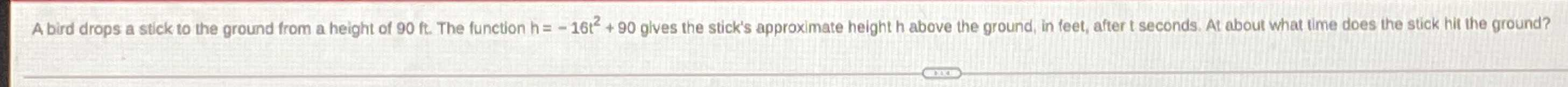### ¿Todavía tienes preguntas de matemáticas?

Pregunte a nuestros tutores expertos
Algebra
PreguntaA bird drops a stick to the ground from a height of $$90 ft$$ . The function $$h = - 16 t ^ { 2 } + 90$$ gives the stick's approximate height $$h$$ above the ground, in feet, after $$t$$ seconds. At about what time does the stick hit the ground?

$$- 16t^{2}+ 90= 0\\t= \sqrt{\frac{90}{16}} \approx 2.4$$